Mar 03, 2023

# Building an FPGA Computer: SAP-3

Previously I built the SAP-1 and SAP-2 in Verilog based on a design laid out in the book Digital Computer Electronics by Malvino and Brown. Now it’s time to build the final evolution of the computer from that book, the SAP-3.

The SAP-1 was very simplistic but very well-explained. The SAP-2 was a big step up from the SAP-1 but explained rather poorly, and it required me to do a lot of awkward things with its design to get it to match the spec laid out in the book. The SAP-3 is even more complex than the SAP-2 but thankfully it didn’t require an awkward design because it’s essentially an Intel 8080/8085 with some instructions removed.

Once again when creating my FPGA version, I decided to be content with it being functionally identical without getting hung up on the implementation details. A program written for the SAP-3 should have the same output when run on my version, but it may finish in more or less time.

This post is intended as a supplement to the first and second so I’ll assume readers of this one are familiar already with the SAP-1 and SAP-2.

## Overview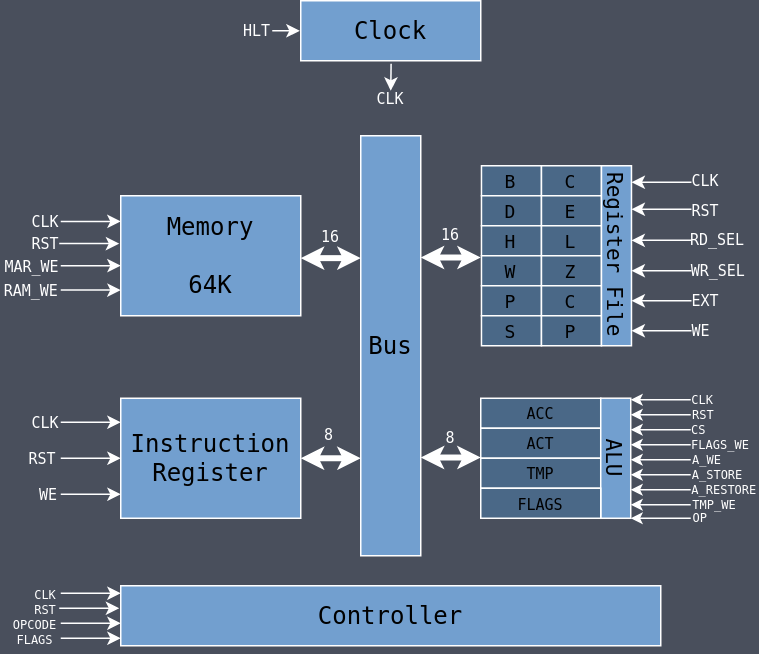The SAP-3 supports more instructions, has more registers, and has a more complicated ALU, but it’s actually more simple than the SAP-2 because of the design of the instructions and how they encode various data (to be explained later).

If you compare this overview architecture diagram to the SAP-2 it looks much simpler with fewer control signals even though it’s a more capable machine.

## Modules

### Clock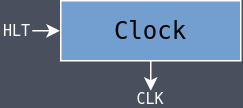The clock is unchanged from the SAP-1 and SAP-2.

 ``````1 2 3 4 5 6 7 8 `````` ``````module clock( input hlt, input clk_in, output clk_out); assign clk_out = (hlt) ? 1'b0 : clk_in; endmodule ``````

### Instruction RegisterThe Instruction Register is unchanged from the SAP-2.

 `````` 1 2 3 4 5 6 7 8 9 10 11 12 13 14 15 16 17 18 19 20 21 `````` ``````module ir( input clk, input rst, input we, input[7:0] bus, output[7:0] out ); reg[7:0] ir; always @(posedge clk, posedge rst) begin if (rst) begin ir <= 8'b0; end else if (we) begin ir <= bus; end end assign out = ir; endmodule ``````

### Register File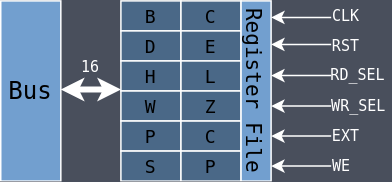The SAP-1 and SAP-2 had discrete register modules while the SAP-3 has a single module called the Register File which contains all of the other registers.

There are six 8-bit programmer-accessible registers available: B, C, D, E, H, and L.

The 8-bit registers can be paired together for some instructions to form three 16-bit registers: BC, DE, and HL.

There are also two dedicated 16-bit registers with special functions: the Program Counter (PC) and the Stack Pointer (SP). They’re always operated on as 16-bits but they’re laid out in Verilog as two 8-bit values.

The PC in the SAP-1 and SAP-2 was its own module but now it’s been brought into the register file.

The SAP-2 had a stack but it was hard-coded to be the two bytes at addresses 0xFFFE and 0xFFFF. The SAP-3 uses SP to point to the address of the bottom of the stack.

There are also two 8-bit programmer-inaccessible registers: W and Z. They’re used as temporary storage for certain instructions. The SAP-2 was missing them which ended up overcomplicating the memory module as it was responsible for holding temporary data in its Memory Data Register.

The registers are laid out in the array called data in a specific order:

``````0000 - B
0001 - C
0010 - D
0011 - E
0100 - H
0101 - L
0110 - W
0111 - Z
1000 - P
1001 - C
1010 - S
1011 - P
``````

I chose that order because, as shown later, all of the instructions encode the registers in that order as well (at least for B-L). That makes it simple to take the bits from the opcode and pass them in directly through the rd_sel and wr_sel inputs. When needing to referencing W, Z, PC, or SP, they’re explicitly enabled.

16-bit increments and decrements (instructions INX and DCX) go through the register file rather than the ALU because they shouldn’t affect the flags. Whether to perform an increment or decrement comes from the ext signal. There is also the ability to do a double increment which is used for skipping over the address bytes of a conditional jump when the condition is not met.

rd_sel and wr_sel are five bits even though only four are needed to encode the twelve registers; bit 5 is set when the register should be treated as the 16-bit extended register. For example, if rd_sel is 10000, then BC should be read.

 `````` 1 2 3 4 5 6 7 8 9 10 11 12 13 14 15 16 17 18 19 20 21 22 23 24 25 26 27 28 29 30 31 32 33 34 35 36 37 38 39 40 41 42 43 44 45 46 47 48 49 50 51 52 53 54 `````` ``````module reg_file( input clk, input rst, input[4:0] rd_sel, input[4:0] wr_sel, input[1:0] ext, input we, input[15:0] data_in, output[15:0] data_out ); reg[7:0] data[0:11]; reg[15:0] data_out; wire wr_ext = wr_sel; wire rd_ext = rd_sel; wire[3:0] wr_dst = wr_sel[3:0]; wire[3:0] rd_src = rd_sel[3:0]; localparam EXT_INC = 2'b01; localparam EXT_DEC = 2'b10; localparam EXT_INC2 = 2'b11; always @(posedge clk, posedge rst) begin if (rst) begin data <= 8'b0; data <= 8'b0; data <= 8'b0; data <= 8'b0; data <= 8'b0; data <= 8'b0; data <= 8'b0; data <= 8'b0; data <= 8'b0; data <= 8'b0; data <= 8'b0; data <= 8'b0; end else begin if (ext == EXT_INC) begin {data[wr_dst], data[wr_dst+1]} <= {data[wr_dst], data[wr_dst+1]} + 1; end else if (ext == EXT_INC2) begin {data[wr_dst], data[wr_dst+1]} <= {data[wr_dst], data[wr_dst+1]} + 2; end else if (ext == EXT_DEC) begin {data[wr_dst], data[wr_dst+1]} <= {data[wr_dst], data[wr_dst+1]} - 1; end else if (we) begin if (wr_ext) begin {data[wr_dst], data[wr_dst+1]} <= data_in; end else begin data[wr_dst] <= data_in[7:0]; end end end end always @(*) begin if (rd_ext) begin data_out = {data[rd_src], data[rd_src+1]}; end else begin data_out = {8'b0, data[rd_src]}; end end endmodule ``````

### ALU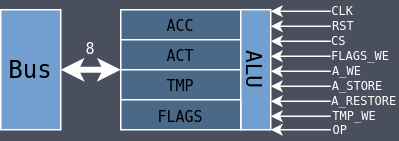The ALU has been expanded significantly from the SAP-2 to support more operations. It has the standard 8-bit accumulator (acc) which is used for all of its operations as well as two temporary registers: act and tmp. When an operation requires two operands, one is acc and the other is tmp. When an operation needs to use the accumulator but doesn’t want to overwrite its value, acc is copied to act and then act is later copied to acc.

The flags register from the SAP-2 has been moved inside of the ALU which is how it is normally done. There are four flags: Zero (Z), Carry (C), Parity (P), and Sign (S). The flags are set differently depending on the operation as some operations should not affect certain flags.

 `````` 1 2 3 4 5 6 7 8 9 10 11 12 13 14 15 16 17 18 19 20 21 22 23 24 25 26 27 28 29 30 31 32 33 34 35 36 37 38 39 40 41 42 43 44 45 46 47 48 49 50 51 52 53 54 55 56 57 58 59 60 61 62 63 64 65 66 67 68 69 70 71 72 73 74 75 76 77 78 79 80 81 82 83 84 85 86 87 88 89 90 91 92 93 94 95 96 97 98 99 100 101 102 103 104 105 106 107 108 109 110 111 112 113 114 115 116 117 118 119 120 121 122 123 124 125 126 127 128 129 130 131 132 133 134 135 136 137 138 139 140 141 142 143 144 145 146 147 148 149 150 151 152 153 154 155 156 157 158 159 160 161 162 163 164 165 166 167 168 169 170 171 172 `````` ``````module alu( input clk, input rst, input cs, input flags_we, input a_we, input a_store, input a_restore, input tmp_we, input[4:0] op, input[7:0] bus, output[7:0] flags, output[7:0] out ); reg carry; wire flg_c; wire flg_z; wire flg_p; wire flg_s; reg[7:0] acc; reg[7:0] flg; reg[7:0] act; reg[7:0] tmp; localparam FLG_Z = 0; localparam FLG_C = 1; localparam FLG_P = 2; localparam FLG_S = 3; localparam OP_ADD = 5'b00000; localparam OP_ADC = 5'b00001; localparam OP_SUB = 5'b00010; localparam OP_SBB = 5'b00011; localparam OP_ANA = 5'b00100; localparam OP_XRA = 5'b00101; localparam OP_ORA = 5'b00110; localparam OP_CMP = 5'b00111; localparam OP_RLC = 5'b01000; localparam OP_RRC = 5'b01001; localparam OP_RAL = 5'b01010; localparam OP_RAR = 5'b01011; localparam OP_DAA = 5'b01100; // Unsupported localparam OP_CMA = 5'b01101; localparam OP_STC = 5'b01110; localparam OP_CMC = 5'b01111; localparam OP_INR = 5'b10000; localparam OP_DCR = 5'b10001; assign flg_c = (carry == 1'b1); assign flg_z = (acc[7:0] == 8'b0); assign flg_s = acc; assign flg_p = ~^acc[7:0]; always @(posedge clk, posedge rst) begin if (rst) begin acc <= 8'b0; act <= 8'b0; tmp <= 8'b0; carry <= 1'b0; end else begin if (a_we) begin acc <= bus; end else if (a_restore) begin acc <= act; end else if (cs) begin case (op) OP_ADD: begin {carry, acc} <= acc + tmp; end OP_ADC: begin {carry, acc} <= acc + tmp + flg[FLG_C]; end OP_SUB: begin {carry, acc} <= acc - tmp; end OP_SBB: begin {carry, acc} <= acc - tmp - flg[FLG_C]; end OP_ANA: begin {carry, acc} <= acc & tmp; end OP_XRA: begin {carry, acc} <= acc ^ tmp; end OP_ORA: begin {carry, acc} <= acc | tmp; end OP_CMP: begin act <= acc - tmp; end OP_RLC: begin carry <= acc; acc <= acc << 1; end OP_RRC: begin carry <= acc; acc <= acc >> 1; end OP_RAL: begin carry <= acc; acc <= (acc << 1 | {7'b0, flg[FLG_C]}); end OP_RAR: begin carry <= acc; acc <= (acc >> 1 | {flg[FLG_C], 7'b0}); end OP_CMA: begin acc <= ~acc; end OP_STC: begin carry <= 1'b1; end OP_CMC: begin carry <= ~flg[FLG_C]; end OP_INR: begin acc <= acc + 1; end OP_DCR: begin acc <= acc - 1; end endcase end if (a_store) act <= acc; if (tmp_we) tmp <= bus; end end always @(negedge clk, posedge rst) begin if (rst) begin flg <= 8'b0; end else if (flags_we) begin flg <= bus; end else begin if (cs) begin case (op) OP_ADD, OP_ADC, OP_SUB, OP_SBB, OP_ANA, OP_XRA, OP_ORA: begin flg[FLG_C] <= flg_c; flg[FLG_Z] <= flg_z; flg[FLG_S] <= flg_s; flg[FLG_P] <= flg_p; end OP_CMP: begin flg[FLG_Z] <= (act == 8'b0); end OP_INR, OP_DCR: begin flg[FLG_Z] <= flg_z; flg[FLG_S] <= flg_s; flg[FLG_P] <= flg_p; end OP_RLC, OP_RRC, OP_RAL, OP_RAR, OP_STC, OP_CMC: begin flg[FLG_C] <= flg_c; end endcase end end end assign flags = flg; assign out = acc; endmodule ``````

### Memory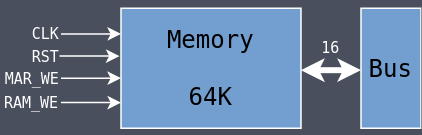The memory module is significantly less complicated this time around. It has the 64K of memory in ram and then a 16-bit Memory Address Register (MAR) to hold addresses that it can then use for reading and writing from memory. No other logic is needed.

 `````` 1 2 3 4 5 6 7 8 9 10 11 12 13 14 15 16 17 18 19 20 21 22 23 24 25 26 27 28 29 30 31 `````` ``````module memory( input clk, input rst, input mar_we, input ram_we, input[15:0] bus, output[7:0] out ); initial begin \$readmemh("program.bin", ram); end reg[15:0] mar; reg[7:0] ram[0:65535]; always @(posedge clk, posedge rst) begin if (rst) mar <= 16'b0; else if (mar_we) mar <= bus; end always @(posedge clk) begin if (ram_we) ram[mar] <= bus[7:0]; end assign out = ram[mar]; endmodule ``````

### Bus

The bus is similar to how it was in SAP-1 and SAP-2 but with different signals. This time there are only four output signals which ever drive the bus: reg_oe, mem_oe, alu_oe, and alu_flags_oe.

 `````` 1 2 3 4 5 6 7 8 9 10 11 12 13 14 `````` ``````reg[15:0] bus; always @(*) begin bus = 16'b0; if (reg_oe) bus = reg_out; else if (mem_oe) bus = {8'b0, mem_out}; else if (alu_oe) bus = {8'b0, alu_out}; else if (alu_flags_oe) bus = {8'b0, alu_flags}; end ``````

### Controller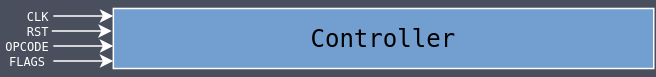The controller for the SAP-1 used a case statement to assert the different control signals depending on the opcode and the current stage of execution. That was possible because there were so few instructions.

The controller for the SAP-2 instead used a control ROM to hold the different signals because it had more instructions and a lot of Verilog would have needed to be written to manage all of the different instructions.

For the SAP-3, I’ve gone back to the case statement approach because, even though there are many more instructions than the SAP-2, they’ve been decoded such that we can extract the relevant bits from the opcode and control the signals in a way that is logical and makes sense.

It still makes for quite a lot of Verilog (nearly 1000 lines) but it’s all much more readable and understandable than trying to fill out a spreadsheet like was done for the SAP-2. It is also likely more inline with how instructions were decoded for the actual Intel 8080/8085.

Rather than listing all of the code here, I’ll talk about it in the dedicated Instruction Decoding section.

## Instruction Set

The SAP-3 has the same instruction set as the Intel 8085 but with some instructions left out. I’ve listed all of the instructions I implemented in the following table, leaving out those not mentioned in the book, while also removing IN and OUT as they aren’t really relevant here. They’ve been ordered by similarity of function rather than alphabetically or by opcode.

The T states shown in the table are the number of cycles required to complete the entire instruction as implemented in my version, which are sometimes higher than the actual 8085 and sometimes lower.

The 8085 has eight flags but the SAP-3 only has four:

• [S]ign   - the instruction resulted in a value less than zero
• [Z]ero   - the instruction resulted in a value of zero
• [P]arity - the instruction resulted in even (0) or odd (1) parity
• [C]arry  - the instruction resulted in a carry bit

### Descriptive Table

InstructionOpcodeBytesFlagsT StatesDescription
INR A3C1SZP-4A = A + 1
INR B041SZP-6B = B + 1
INR C0C1SZP-6C = C + 1
INR D141SZP-6D = D + 1
INR E1C1SZP-6E = E + 1
INR H241SZP-6H = H + 1
INR L2C1SZP-6L = L + 1
INR M341SZP-7[HL] = [HL] + 1
DCR A3D1SZP-4A = A - 1
DCR B051SZP-6B = B - 1
DCR C0D1SZP-6C = C - 1
DCR D151SZP-6D = D - 1
DCR E1D1SZP-6E = E - 1
DCR H251SZP-6H = H - 1
DCR L2D1SZP-6L = L - 1
DCR M351SZP-7[HL] = [HL] - 1
INX B031----4BC = BC + 1
INX D131----4DE = DE + 1
INX H231----4HL = HL + 1
INX SP331----4SP = SP + 1
DCX B0B1----4BC = BC - 1
DCX D1B1----4DE = DE - 1
DCX H2B1----4HL = HL - 1
DCX SP3B1----4SP = SP - 1
DAD B091---C12HL = HL + BC
DAD D191---C12HL = HL + DE
DAD H291---C12HL = HL + HL
DAD SP391---C12HL = HL + SP
ADD A871SZPC4A = A + A
ADD B801SZPC5A = A + B
ADD C811SZPC5A = A + C
ADD D821SZPC5A = A + D
ADD E831SZPC5A = A + E
ADD H841SZPC5A = A + H
ADD L851SZPC5A = A + L
ADD M861SZPC6A = A + [HL]
ADI byteC62SZPC6A = A + byte
ADC A8F1SZPC4A = A + A + FlagC
ADC B881SZPC5A = A + B + FlagC
ADC C891SZPC5A = A + C + FlagC
ADC D8A1SZPC5A = A + D + FlagC
ADC E8B1SZPC5A = A + E + FlagC
ADC H8C1SZPC5A = A + H + FlagC
ADC L8D1SZPC5A = A + L + FlagC
ADC M8E1SZPC6A = A + [HL] + FlagC
ACI byteCE2SZPC6A = A + byte + FlagC
SUB A971SZPC4A = A - A
SUB B901SZPC5A = A - B
SUB C911SZPC5A = A - C
SUB D921SZPC5A = A - D
SUB E931SZPC5A = A - E
SUB H941SZPC5A = A - H
SUB L951SZPC5A = A - L
SUB M961SZPC6A = A - [HL]
SUI byteD62SZPC6A = A - byte
SBB A9F1SZPC4A = A - byte - FlagC
SBB B981SZPC5A = A - byte - FlagC
SBB C991SZPC5A = A - byte - FlagC
SBB D9A1SZPC5A = A - byte - FlagC
SBB E9B1SZPC5A = A - byte - FlagC
SBB H9C1SZPC5A = A - byte - FlagC
SBB L9D1SZPC5A = A - byte - FlagC
SBB M9E1SZPC6A = A - byte - FlagC
SBI byteDE2SZPC6A = A - byte - FlagC
ANA AA71SZPC4A = A and A
ANA BA01SZPC5A = A and B
ANA CA11SZPC5A = A and C
ANA DA21SZPC5A = A and D
ANA EA31SZPC5A = A and E
ANA HA41SZPC5A = A and H
ANA LA51SZPC5A = A and L
ANA MA61SZPC6A = A and [HL]
ANI byteE62SZPC6A = A and byte
ORA AB71SZPC4A = A or A
ORA BB01SZPC5A = A or B
ORA CB11SZPC5A = A or C
ORA DB21SZPC5A = A or D
ORA EB31SZPC5A = A or E
ORA HB41SZPC5A = A or H
ORA LB51SZPC5A = A or L
ORA MB61SZPC6A = A or [HL]
ORI byteF62SZPC6A = A or byte
XRA AAF1SZPC4A = A xor A
XRA BA81SZPC5A = A xor B
XRA CA91SZPC5A = A xor C
XRA DAA1SZPC5A = A xor D
XRA EAB1SZPC5A = A xor E
XRA HAC1SZPC5A = A xor H
XRA LAD1SZPC5A = A xor L
XRA MAE1SZPC6A = A xor [HL]
XRI byteEE2SZPC6A = A xor byte
RLC071---C4Shift A left and FlagC = A
RAL171---C4Shift A left and shift FlagC into A
RAR1F1---C4Shift A right and shift FlagC into A
RRC0F1---C4Shift A right and FlagC = A
CMA2F1----4A = ~A
STC371---C4FlagC = 1
CMC3F1---C4FlagC = ~FlagC
CMP ABF1SZPC4FlagZ = 1 if A == A
CMP BB81SZPC5FlagZ = 1 if A == B
CMP CB91SZPC5FlagZ = 1 if A == C
CMP DBA1SZPC5FlagZ = 1 if A == D
CMP EBB1SZPC5FlagZ = 1 if A == E
CMP HBC1SZPC5FlagZ = 1 if A == H
CMP LBD1SZPC5FlagZ = 1 if A == L
CMP MBE1SZPC6FlagZ = 1 if A == [HL]
CPI byteFE2----6FlagZ = 1 if A == byte
LXI B, dble013----10Load BC with dble
LXI D, dble113----10Load DE with dble
LXI H, dble213----10Load HL with dble
LXI SP, dble313----10Load SP with dble
STAX B021----8Store A at [BC]
STAX D121----8Store A at [DE]
MOV A, A7F1----4A = A
MOV A, B781----4A = B
MOV A, C791----4A = C
MOV A, D7A1----4A = D
MOV A, E7B1----4A = E
MOV A, H7C1----4A = H
MOV A, L7D1----4A = L
MOV A, M7E1----5A = [HL]
MOV B, A471----4B = A
MOV B, B401----4B = B
MOV B, C411----4B = C
MOV B, D421----4B = D
MOV B, E431----4B = E
MOV B, H441----4B = H
MOV B, L451----4B = L
MOV B, M461----5B = [HL]
MOV C, A4F1----4C = A
MOV C, B481----4C = B
MOV C, C491----4C = C
MOV C, D4A1----4C = D
MOV C, E4B1----4C = E
MOV C, H4C1----4C = H
MOV C, L4D1----4C = L
MOV C, M4E1----5C = [HL]
MOV D, A571----4D = A
MOV D, B501----4D = B
MOV D, C511----4D = C
MOV D, D521----4D = D
MOV D, E531----4D = E
MOV D, H541----4D = H
MOV D, L551----4D = L
MOV D, M561----5D = [HL]
MOV E, A5F1----4E = A
MOV E, B581----4E = B
MOV E, C591----4E = C
MOV E, D5A1----4E = D
MOV E, E5B1----4E = E
MOV E, H5C1----4E = H
MOV E, L5D1----4E = L
MOV E, M5E1----5E = [HL]
MOV H, A671----4H = A
MOV H, B601----4H = B
MOV H, C611----4H = C
MOV H, D621----4H = D
MOV H, E631----4H = E
MOV H, H641----4H = H
MOV H, L651----4H = L
MOV H, M661----5H = [HL]
MOV L, A6F1----4L = A
MOV L, B681----4L = B
MOV L, C691----4L = C
MOV L, D6A1----4L = D
MOV L, E6B1----4L = E
MOV L, H6C1----4L = H
MOV L, L6D1----4L = L
MOV L, M6E1----5L = [HL]
MOV M, A771----5[HL] = A
MOV M, B701----5[HL] = B
MOV M, C711----5[HL] = C
MOV M, D721----5[HL] = D
MOV M, E731----5[HL] = E
MOV M, H741----5[HL] = H
MOV M, L751----5[HL] = L
MVI A, byte3E2----6A = byte
MVI B, byte062----6B = byte
MVI C, byte0E2----6C = byte
MVI D, byte162----6D = byte
MVI E, byte1E2----6E = byte
MVI H, byte262----6H = byte
MVI L, byte2E2----6L = byte
MVI M, byte362----8[HL] = byte
PUSH BC51SZPC9Push value in BC onto the stack
PUSH DD51SZPC9Push value in DE onto the stack
PUSH HE51SZPC9Push value in HL onto the stack
PUSH PSWF51SZPC9Push value in AF onto the stack
POP BC11SZPC9Pop value on stack into BC
POP DD11SZPC9Pop value on stack into DE
POP HE11SZPC9Pop value on stack into HL
POP PSWF11SZPC9Pop value on stack into AF
RETC91----4Return from function
RPF01----4/10Return from function if FlagS == 0
RMF81----4/10Return from function if FlagS == 1
RNZC01----4/10Return from function if FlagZ == 0
RZC81----4/10Return from function if FlagZ == 1
RPOE01----4/10Return from function if FlagP == 0
RPEE81----4/10Return from function if FlagP == 1
RNCD01----4/10Return from function if FlagC == 0
RCD81----4/10Return from function if FlagC == 1
NOP001----4Do nothing
HLT761----4Halt execution

All of the opcodes may look random at first but if you arrange them by their opcodes then it begins to make a little more sense: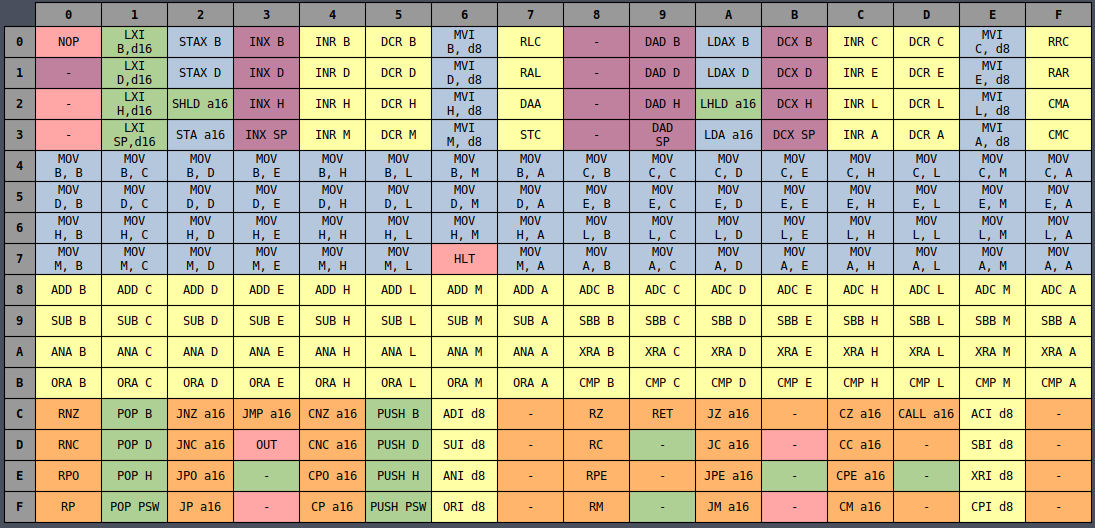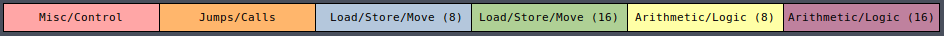It’s clear from the color coding that there’s definitely a designed ordering to all of the instructions such that similar instructions are (mostly) laid out near each other. But it still seems slightly random. Why are half of the MVI instructions on the left side and the other half on the right side? Why are INR and DCR split in half but still next to each other?

### Octal Table

It all becomes much more clear if you instead view the table in octal representation: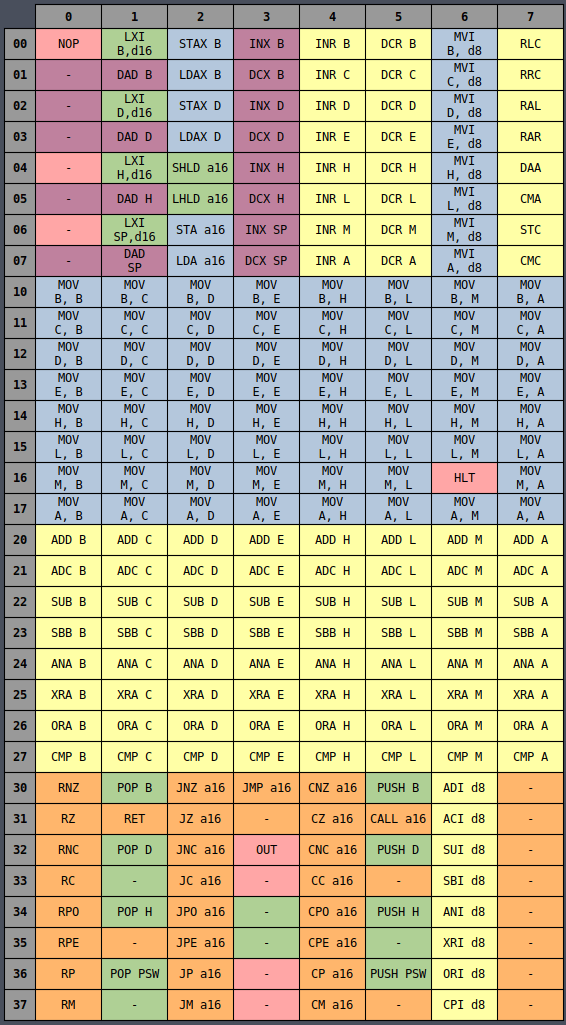Now all of the MVI instructions are in a single column and the same is true for INR, DCR, and many others, whether they’re sorted by column or row. There is an underlying logic to all of the opcodes which is very useful later on for wiring up the controller logic.

## Instruction Decoding

The way the decoding works is that different bits of the opcodes mean different things depending on the type of instruction being decoded. The pattern to the bits makes it easier to decode them into control signals. Rather than doing checks against every single opcode and then setting the required signals by hand (like was done for SAP-1 and SAP-2), instead we can pull bits out of the opcode and connect them to signals without needing to care what the bits actually are.

In a Verilog casez statement, you can specify certain bits as Don’t Care (?) which means that the case statement will match any bit pattern satisfying the other bits while not caring if the ? bits match or not. For example, the 8-bit octal pattern 8’o1?6 will match: 8’o106, 8’o116, 8’o126, 8’o136, 8’o146, 8’o156, 8’o166, and 8’o176.

Case statements listed earlier will override those listed later, so if you first have 8’o1?6 and then later 8’o1??, the first will match before the second, allowing it override specific cases you care about.

There are probably smarter ways of condensing the case statements even further based on the encoding, but I didn’t want to go so far with it that it became hard to read them.

The first three stages are always the same: grab the next instruction from memory and write it into the program counter. Then stages four and beyond do different things depending on the instruction.

The framework for the instruction decoding in the controller looks like this:

 `````` 1 2 3 4 5 6 7 8 9 10 11 12 13 14 15 16 17 18 19 20 21 22 23 24 25 26 27 28 29 30 31 32 33 34 35 36 37 38 39 40 41 42 43 44 45 46 47 48 `````` ``````module controller( input clk, input rst, input[7:0] opcode, input[7:0] flags, output[32:0] out ); reg[32:0] ctrl_word; reg[3:0] stage; reg stage_rst; assign out = ctrl_word; always @(negedge clk, posedge rst) begin if (rst) begin stage <= 0; end else begin if (stage_rst) begin stage <= 0; end else begin stage <= stage + 1; end end end always @(*) begin ctrl_word = 0; stage_rst = 0; if (stage == 0) begin ctrl_word[REG_RD_SEL4:REG_RD_SEL0] = REG_PC; ctrl_word[REG_OE] = 1'b1; ctrl_word[MEM_MAR_WE] = 1'b1; end else if (stage == 1) begin ctrl_word[MEM_OE] = 1'b1; ctrl_word[IR_WE] = 1'b1; end else if (stage == 2) begin ctrl_word[REG_WR_SEL4:REG_WR_SEL0] = REG_PC; ctrl_word[REG_EXT1:REG_EXT0] = REG_INC; end else begin casez (opcode) // ... // ... // ... endcase end end ``````

From here I’ll explain the way each set of related instructions are decoded and show how they map to Verilog.

### MOV Rd, Rs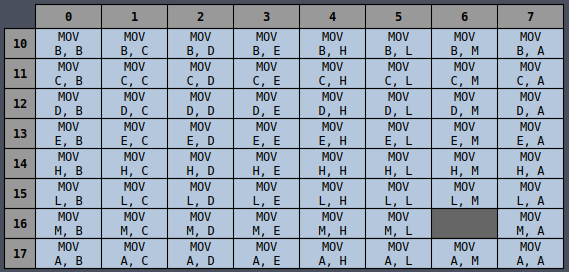Pattern: 8’o1??

opcode[5:3] - Rd

• 000 - B
• 001 - C
• 010 - D
• 011 - E
• 100 - H
• 101 - L
• 110 - M
• 111 - A

opcode[2:0] - Rs

• 000 - B
• 001 - C
• 010 - D
• 011 - E
• 100 - H
• 101 - L
• 110 - M
• 111 - A

The control signals are different when the source or destination register is M, so they have their own separate cases that come first to override the later general case.

 `````` 1 2 3 4 5 6 7 8 9 10 11 12 13 14 15 16 17 18 19 20 21 22 23 24 25 26 27 28 29 30 31 32 33 34 35 36 37 38 39 40 41 42 43 44 45 46 47 48 49 50 51 52 53 54 55 56 57 58 59 60 61 62 `````` ``````// MOV Rd, M // opcode[5:3] - Rd 8'o1?6: begin if (stage == 3) begin ctrl_word[REG_RD_SEL4:REG_RD_SEL0] = REG_HL; ctrl_word[REG_OE] = 1'b1; ctrl_word[MEM_MAR_WE] = 1'b1; end else if (stage == 4) begin if (opcode[5:3] == 3'b111) begin ctrl_word[ALU_A_WE] = 1'b1; end else begin ctrl_word[REG_WR_SEL4:REG_WR_SEL0] = {2'b0, opcode[5:3]}; ctrl_word[REG_WE] = 1'b1; end ctrl_word[MEM_OE] = 1'b1; stage_rst = 1'b1; end end // MOV M, Rs // opcode[2:0] - Rs 8'o16?: begin if (stage == 3) begin ctrl_word[REG_RD_SEL4:REG_RD_SEL0] = REG_HL; ctrl_word[REG_OE] = 1'b1; ctrl_word[MEM_MAR_WE] = 1'b1; end else if (stage == 4) begin if (opcode[2:0] == 3'b111) begin ctrl_word[ALU_OE] = 1'b1; end else begin ctrl_word[REG_RD_SEL4:REG_RD_SEL0] = {2'b0, opcode[2:0]}; ctrl_word[REG_OE] = 1'b1; end ctrl_word[MEM_WE] = 1'b1; stage_rst = 1'b1; end end // MOV Rd, Rs // opcode[5:3] - Rd // opcode[2:0] - Rs 8'o1??: begin if (stage == 3) begin if (opcode[2:0] == 3'b111) begin ctrl_word[ALU_OE] = 1'b1; end else begin ctrl_word[REG_RD_SEL4:REG_RD_SEL0] = {2'b0, opcode[2:0]}; ctrl_word[REG_OE] = 1'b1; end if (opcode[5:3] == 3'b111) begin ctrl_word[ALU_A_WE] = 1'b1; end else begin ctrl_word[REG_WR_SEL4:REG_WR_SEL0] = {2'b0, opcode[5:3]}; ctrl_word[REG_WE] = 1'b1; end stage_rst = 1'b1; end end ``````

### MOV Immediate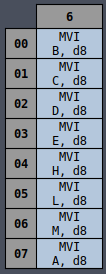Pattern: 8’o0?6

opcode[5:3] - Rd

• 000 - B
• 001 - C
• 010 - D
• 011 - E
• 100 - H
• 101 - L
• 110 - M
• 111 - A

The control signals are different when the destination register is M, so it has its own separate case that come first to override the later general case.

 `````` 1 2 3 4 5 6 7 8 9 10 11 12 13 14 15 16 17 18 19 20 21 22 23 24 25 26 27 28 29 30 31 32 33 34 35 36 37 38 39 40 41 42 43 44 45 46 47 `````` ``````// MVI M, d8 8'o066: begin if (stage == 3) begin ctrl_word[REG_RD_SEL4:REG_RD_SEL0] = REG_PC; ctrl_word[REG_OE] = 1'b1; ctrl_word[MEM_MAR_WE] = 1'b1; end else if (stage == 4) begin ctrl_word[MEM_OE] = 1'b1; ctrl_word[REG_WR_SEL4:REG_WR_SEL0] = {2'b0, REG_WZ_W}; ctrl_word[REG_WE] = 1'b1; end else if (stage == 5) begin ctrl_word[REG_RD_SEL4:REG_RD_SEL0] = REG_HL; ctrl_word[REG_OE] = 1'b1; ctrl_word[MEM_MAR_WE] = 1'b1; end else if (stage == 6) begin ctrl_word[REG_RD_SEL4:REG_RD_SEL0] = {2'b0, REG_WZ_W}; ctrl_word[REG_OE] = 1'b1; ctrl_word[MEM_WE] = 1'b1; end else if (stage == 7) begin ctrl_word[REG_WR_SEL4:REG_WR_SEL0] = REG_PC; ctrl_word[REG_EXT1:REG_EXT0] = REG_INC; stage_rst = 1'b1; end end // MVI Rd, d8 // opcode[5:3] - Rd 8'o0?6: begin if (stage == 3) begin ctrl_word[REG_RD_SEL4:REG_RD_SEL0] = REG_PC; ctrl_word[REG_OE] = 1'b1; ctrl_word[MEM_MAR_WE] = 1'b1; end else if (stage == 4) begin if (opcode[5:3] == 3'b111) begin ctrl_word[ALU_A_WE] = 1'b1; end else begin ctrl_word[REG_WR_SEL4:REG_WR_SEL0] = {2'b0, opcode[5:3]}; ctrl_word[REG_WE] = 1'b1; end ctrl_word[MEM_OE] = 1'b1; end else if (stage == 5) begin ctrl_word[REG_WR_SEL4:REG_WR_SEL0] = REG_PC; ctrl_word[REG_EXT1:REG_EXT0] = REG_INC; stage_rst = 1'b1; end end ``````

### Increment / Decrement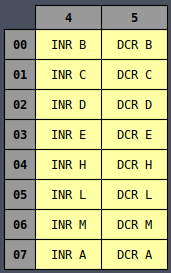Pattern: 8’o0?4, 8’o0?5

opcode

• 0 - Increment (INR)
• 1 - Decrement (DCR)

opcode[5:3]

• 000 - B
• 001 - C
• 010 - D
• 011 - E
• 100 - H
• 101 - L
• 110 - M
• 111 - A

The control signals are different when the source register is M, so it has its own separate case that come first to override the later general case.

 `````` 1 2 3 4 5 6 7 8 9 10 11 12 13 14 15 16 17 18 19 20 21 22 23 24 25 26 27 28 29 30 31 32 33 34 35 36 37 38 39 40 41 42 43 44 45 46 47 48 49 `````` ``````// INR/DCR M // opcode[5:3] - Rs // opcode - INR (0), DCR (1) 8'o064: begin if (stage == 3) begin ctrl_word[REG_RD_SEL4:REG_RD_SEL0] = REG_HL; ctrl_word[REG_OE] = 1'b1; ctrl_word[MEM_MAR_WE] = 1'b1; end else if (stage == 4) begin ctrl_word[MEM_OE] = 1'b1; ctrl_word[ALU_A_STORE] = 1'b1; ctrl_word[ALU_A_WE] = 1'b1; end else if (stage == 5) begin ctrl_word[ALU_CS] = 1'b1; ctrl_word[ALU_OP4:ALU_OP0] = {4'b1000, opcode}; end else if (stage == 6) begin ctrl_word[ALU_OE] = 1'b1; ctrl_word[ALU_A_RESTORE] = 1'b1; ctrl_word[MEM_WE] = 1'b1; stage_rst = 1'b1; end end // INR/DCR Rs // opcode[5:3] - Rs // opcode - INR (0), DCR (1) 8'o0?4, 8'o0?5: begin if (stage == 3) begin if (opcode[5:3] == 3'b111) begin ctrl_word[ALU_CS] = 1'b1; ctrl_word[ALU_OP4:ALU_OP0] = 5'b10000; stage_rst = 1'b1; end else begin ctrl_word[REG_RD_SEL4:REG_RD_SEL0] = {2'b0, opcode[5:3]}; ctrl_word[REG_OE] = 1'b1; ctrl_word[ALU_A_STORE] = 1'b1; ctrl_word[ALU_A_WE] = 1'b1; end end else if (stage == 4) begin ctrl_word[ALU_CS] = 1'b1; ctrl_word[ALU_OP4:ALU_OP0] = {4'b1000, opcode}; end else if (stage == 5) begin ctrl_word[ALU_OE] = 1'b1; ctrl_word[ALU_A_RESTORE] = 1'b1; ctrl_word[REG_WR_SEL4:REG_WR_SEL0] = {2'b0, opcode[5:3]}; ctrl_word[REG_WE] = 1'b1; stage_rst = 1'b1; end end ``````

### Increment / Decrement (16-bit)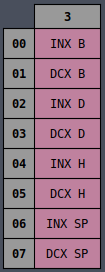Pattern: 8’o0?3

opcode

• 0 - Increment (INX)
• 1 - Decrement (DCX)

opcode[5:4]

• 00 - B
• 01 - D
• 10 - HL
• 11 - SP
 `````` 1 2 3 4 5 6 7 8 9 10 11 12 13 14 `````` ``````// INX, DCX // opcode[5:4] - Rs (16-bit) // opcode - Dec(1) / Inc (0) 8'o0?3: begin if (stage == 3) begin if (opcode[5:4] == 2'b11) ctrl_word[REG_WR_SEL4:REG_WR_SEL0] = REG_SP; else ctrl_word[REG_WR_SEL4:REG_WR_SEL0] = {2'b10, opcode[5:4], 1'b0}; ctrl_word[REG_EXT1:REG_EXT0] = {opcode, ~opcode}; stage_rst = 1'b1; end end ``````

### ALU Primary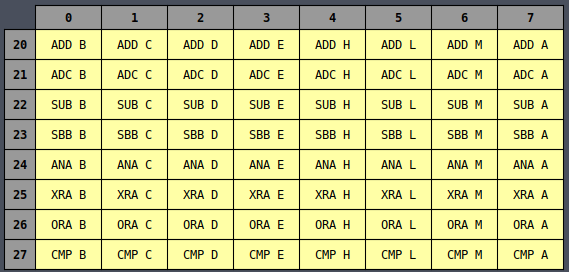Pattern: 8’o2??

opcode[5:3]

• 010 - SUB
• 011 - SBB
• 100 - ANA
• 101 - XRA
• 110 - ORA
• 111 - CMP

opcode[2:0]

• 000 - B
• 001 - C
• 010 - D
• 011 - E
• 100 - H
• 101 - L
• 110 - M
• 111 - A

The control signals are different when the register is M, so it has its own separate case that come first to override the later general case.

 `````` 1 2 3 4 5 6 7 8 9 10 11 12 13 14 15 16 17 18 19 20 21 22 23 24 25 26 27 28 29 30 31 32 33 34 35 36 37 38 `````` ``````// Arithmetic/Logic Set 0 (M) // opcode[5:3] - ALU Op 8'o2?6: begin if (stage == 3) begin ctrl_word[REG_RD_SEL4:REG_RD_SEL0] = REG_HL; ctrl_word[REG_OE] = 1'b1; ctrl_word[MEM_MAR_WE] = 1'b1; end else if (stage == 4) begin ctrl_word[MEM_OE] = 1'b1; ctrl_word[ALU_TMP_WE] = 1'b1; end else if (stage == 5) begin ctrl_word[ALU_CS] = 1'b1; ctrl_word[ALU_OP4:ALU_OP0] = {2'b0, opcode[5:3]}; stage_rst = 1'b1; end end // Arithmetic/Logic Set 0 // opcode[2:0] - Rs // opcode[5:3] - ALU Op 8'o2??: begin if (stage == 3) begin if (opcode[2:0] == 3'b111) begin ctrl_word[ALU_CS] = 1'b1; ctrl_word[ALU_OP4:ALU_OP0] = {2'b0, opcode[5:3]}; stage_rst = 1'b1; end else begin ctrl_word[REG_RD_SEL4:REG_RD_SEL0] = {2'b0, opcode[2:0]}; ctrl_word[REG_OE] = 1'b1; end ctrl_word[ALU_TMP_WE] = 1'b1; end else if (stage == 4) begin ctrl_word[ALU_CS] = 1'b1; ctrl_word[ALU_OP4:ALU_OP0] = {2'b0, opcode[5:3]}; stage_rst = 1'b1; end end ``````

### ALU Secondary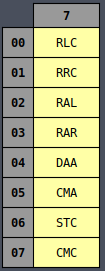Pattern: 8’o0?7

opcode[5:4]

• 000 - RLC
• 001 - RRC
• 010 - RAL
• 011 - RAR
• 100 - DAA
• 101 - CMA
• 110 - STC
• 111 - CMC
 ``````1 2 3 4 5 6 7 8 9 `````` ``````// Arithmetic/Logic Set 1 // opcode[5:3] - ALU Op 8'o0?7: begin if (stage == 3) begin ctrl_word[ALU_CS] = 1'b1; ctrl_word[ALU_OP4:ALU_OP0] = {2'b01, opcode[5:3]}; stage_rst = 1'b1; end end ``````

### ALU Immediate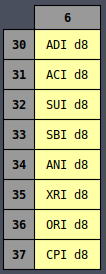Pattern: 8’o3?6

opcode[5:4]

• 001 - ACI
• 010 - SUI
• 011 - SBI
• 100 - ANI
• 101 - XRI
• 110 - ORI
• 111 - CPI
 `````` 1 2 3 4 5 6 7 8 9 10 11 12 13 14 15 16 17 18 `````` ``````// Arithmetic/Logic Immediate // opcode[5:3] - ALU Op 8'o3?6: begin if (stage == 3) begin ctrl_word[REG_RD_SEL4:REG_RD_SEL0] = REG_PC; ctrl_word[REG_OE] = 1'b1; ctrl_word[MEM_MAR_WE] = 1'b1; end else if (stage == 4) begin ctrl_word[MEM_OE] = 1'b1; ctrl_word[ALU_TMP_WE] = 1'b1; end else if (stage == 5) begin ctrl_word[ALU_CS] = 1'b1; ctrl_word[ALU_OP4:ALU_OP0] = {2'b0, opcode[5:3]}; ctrl_word[REG_WR_SEL4:REG_WR_SEL0] = REG_PC; ctrl_word[REG_EXT1:REG_EXT0] = REG_INC; stage_rst = 1'b1; end end ``````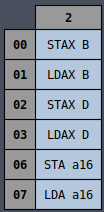Pattern: 8’o002, 8’o010, 8’o022, 8’o032, 8’o062, 8’o072

opcode

• 0 - STA(X)
• 1 - LDA(X)

opcode[5:4]

• 00 - B
• 01 - D
• 11 - a16
 `````` 1 2 3 4 5 6 7 8 9 10 11 12 13 14 15 16 17 18 19 20 21 22 23 24 25 26 27 28 29 30 31 32 33 34 35 36 37 38 39 40 41 42 43 44 45 46 47 48 49 50 51 52 53 54 55 56 57 58 59 60 61 62 63 64 65 66 67 68 69 70 71 72 `````` ``````// STA/LDA a16 // opcode: STA (0) / LDA (1) 8'o062, 8'o072: begin if (stage == 3) begin ctrl_word[REG_RD_SEL4:REG_RD_SEL0] = REG_PC; ctrl_word[REG_OE] = 1'b1; ctrl_word[MEM_MAR_WE] = 1'b1; end else if (stage == 4) begin ctrl_word[MEM_OE] = 1'b1; ctrl_word[REG_WE] = 1'b1; ctrl_word[REG_WR_SEL4:REG_WR_SEL0] = REG_WZ_Z; end else if (stage == 5) begin ctrl_word[REG_WR_SEL4:REG_WR_SEL0] = REG_PC; ctrl_word[REG_EXT1:REG_EXT0] = REG_INC; end else if (stage == 6) begin ctrl_word[REG_RD_SEL4:REG_RD_SEL0] = REG_PC; ctrl_word[REG_OE] = 1'b1; ctrl_word[MEM_MAR_WE] = 1'b1; end else if (stage == 7) begin ctrl_word[MEM_OE] = 1'b1; ctrl_word[REG_WE] = 1'b1; ctrl_word[REG_WR_SEL4:REG_WR_SEL0] = REG_WZ_W; end else if (stage == 8) begin ctrl_word[REG_WR_SEL4:REG_WR_SEL0] = REG_PC; ctrl_word[REG_EXT1:REG_EXT0] = REG_INC; end else if (stage == 9) begin ctrl_word[REG_RD_SEL4:REG_RD_SEL0] = REG_WZ; ctrl_word[REG_OE] = 1'b1; ctrl_word[MEM_MAR_WE] = 1'b1; end else if (stage == 10) begin if (opcode == 0) begin ctrl_word[ALU_OE] = 1'b1; ctrl_word[MEM_WE] = 1'b1; end else begin ctrl_word[ALU_A_WE] = 1'b1; ctrl_word[MEM_OE] = 1'b1; end stage_rst = 1'b1; end end // STAX/LDAX Rs // opcode[5:4] - Rs // opcode - STAX (0) / LDAX (1) 8'o002, 8'o012, 8'o022, 8'o032: begin if (stage == 3) begin ctrl_word[REG_RD_SEL4:REG_RD_SEL0] = {2'b0, opcode[5:4], 1'b0}; ctrl_word[REG_OE] = 1'b1; ctrl_word[REG_WR_SEL4:REG_WR_SEL0] = REG_WZ_W; ctrl_word[REG_WE] = 1'b1; end else if (stage == 4) begin ctrl_word[REG_RD_SEL4:REG_RD_SEL0] = {2'b0, opcode[5:4], 1'b1}; ctrl_word[REG_OE] = 1'b1; ctrl_word[REG_WR_SEL4:REG_WR_SEL0] = REG_WZ_Z; ctrl_word[REG_WE] = 1'b1; end else if (stage == 5) begin ctrl_word[REG_RD_SEL4:REG_RD_SEL0] = REG_WZ; ctrl_word[REG_OE] = 1'b1; ctrl_word[MEM_MAR_WE] = 1'b1; end else if (stage == 7) begin if (opcode == 1'b0) begin ctrl_word[ALU_OE] = 1'b1; ctrl_word[MEM_WE] = 1'b1; end else begin ctrl_word[ALU_A_WE] = 1'b1; ctrl_word[MEM_OE] = 1'b1; end stage_rst = 1'b1; end end ``````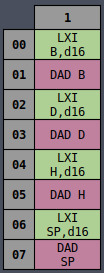Pattern: 8’o0?1

opcode

• 0 - LXI

opcode[5:4]

• 00 - B
• 01 - D
• 10 - HL
• 11 - SP
 `````` 1 2 3 4 5 6 7 8 9 10 11 12 13 14 15 16 17 18 19 20 21 22 23 24 25 26 27 28 29 30 31 32 33 34 35 36 37 38 39 40 41 42 43 44 45 46 47 48 49 50 51 52 53 54 55 56 57 58 59 60 61 62 63 64 65 66 67 68 69 70 71 72 73 74 75 76 77 78 79 80 81 82 83 84 85 86 87 88 89 90 91 `````` ``````// LXI // opcode[5:4] - Extended Register 8'o001, 8'o021, 8'o041, 8'o061: begin if (stage == 3) begin ctrl_word[REG_RD_SEL4:REG_RD_SEL0] = REG_PC; ctrl_word[REG_OE] = 1'b1; ctrl_word[MEM_MAR_WE] = 1'b1; end else if (stage == 4) begin ctrl_word[MEM_OE] = 1'b1; ctrl_word[REG_WE] = 1'b1; ctrl_word[REG_WR_SEL4:REG_WR_SEL0] = REG_WZ_Z; end else if (stage == 5) begin ctrl_word[REG_WR_SEL4:REG_WR_SEL0] = REG_PC; ctrl_word[REG_EXT1:REG_EXT0] = REG_INC; end else if (stage == 6) begin ctrl_word[REG_RD_SEL4:REG_RD_SEL0] = REG_PC; ctrl_word[REG_OE] = 1'b1; ctrl_word[MEM_MAR_WE] = 1'b1; end else if (stage == 7) begin ctrl_word[MEM_OE] = 1'b1; ctrl_word[REG_WE] = 1'b1; ctrl_word[REG_WR_SEL4:REG_WR_SEL0] = REG_WZ_W; end else if (stage == 8) begin if (opcode[5:4] == 2'b11) ctrl_word[REG_WR_SEL4:REG_WR_SEL0] = REG_SP; else ctrl_word[REG_WR_SEL4:REG_WR_SEL0] = {2'b10, opcode[5:4], 1'b0}; ctrl_word[REG_RD_SEL4:REG_RD_SEL0] = REG_WZ; ctrl_word[REG_OE] = 1'b1; ctrl_word[REG_WE] = 1'b1; end else if (stage == 9) begin ctrl_word[REG_WR_SEL4:REG_WR_SEL0] = REG_PC; ctrl_word[REG_EXT1:REG_EXT0] = REG_INC; stage_rst = 1'b1; end end // DAD // opcode[5:4] - Extended Register 8'o011, 8'o031, 8'o051, 8'o071: begin if (stage == 3) begin ctrl_word[REG_RD_SEL4:REG_RD_SEL0] = REG_HL_L; ctrl_word[REG_OE] = 1'b1; ctrl_word[ALU_A_STORE] = 1'b1; ctrl_word[ALU_A_WE] = 1'b1; end else if (stage == 4) begin if (opcode[5:4] == 2'b11) begin ctrl_word[REG_RD_SEL4:REG_RD_SEL0] = REG_SP_P; end else begin ctrl_word[REG_RD_SEL4:REG_RD_SEL0] = {2'b0, opcode[5:4], 1'b1}; end ctrl_word[REG_OE] = 1'b1; ctrl_word[ALU_TMP_WE] = 1'b1; end else if (stage == 5) begin ctrl_word[ALU_CS] = 1'b1; ctrl_word[ALU_OP4:ALU_OP0] = 5'b00000; // Add end else if (stage == 6) begin ctrl_word[REG_WR_SEL4:REG_WR_SEL0] = REG_WZ_Z; ctrl_word[REG_WE] = 1'b1; ctrl_word[ALU_OE] = 1'b1; end else if (stage == 7) begin ctrl_word[REG_RD_SEL4:REG_RD_SEL0] = REG_HL_H; ctrl_word[REG_OE] = 1'b1; ctrl_word[ALU_A_WE] = 1'b1; end else if (stage == 8) begin if (opcode[5:4] == 2'b11) begin ctrl_word[REG_RD_SEL4:REG_RD_SEL0] = REG_SP_S; end else begin ctrl_word[REG_RD_SEL4:REG_RD_SEL0] = {2'b0, opcode[5:4], 1'b0}; end ctrl_word[REG_OE] = 1'b1; ctrl_word[ALU_TMP_WE] = 1'b1; end else if (stage == 9) begin ctrl_word[ALU_CS] = 1'b1; ctrl_word[ALU_OP4:ALU_OP0] = 5'b00001; // Add w/ Carry end else if (stage == 10) begin ctrl_word[REG_WR_SEL4:REG_WR_SEL0] = REG_WZ_W; ctrl_word[REG_WE] = 1'b1; ctrl_word[ALU_OE] = 1'b1; ctrl_word[ALU_A_RESTORE] = 1'b1; end else if (stage == 11) begin ctrl_word[REG_WR_SEL4:REG_WR_SEL0] = REG_HL; ctrl_word[REG_WE] = 1'b1; ctrl_word[REG_RD_SEL4:REG_RD_SEL0] = REG_WZ; ctrl_word[REG_OE] = 1'b1; stage_rst = 1'b1; end end ``````

### Jump / Call / Ret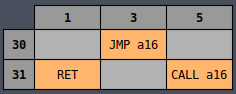There is no great magic to the way these are encoded (at least none that I could find) so they’re checked for explicitly.

 `````` 1 2 3 4 5 6 7 8 9 10 11 12 13 14 15 16 17 18 19 20 21 22 23 24 25 26 27 28 29 30 31 32 33 34 35 36 37 38 39 40 41 42 43 44 45 46 47 48 49 50 51 52 53 54 55 56 57 58 59 60 61 62 63 64 65 66 67 68 69 70 71 72 73 74 75 76 77 78 79 80 81 82 83 84 85 86 87 88 89 90 91 92 93 94 95 96 97 98 99 100 101 102 103 104 105 106 107 108 109 110 111 112 113 114 115 116 117 `````` ``````// JMP 8'o303: begin if (stage == 3) begin ctrl_word[REG_RD_SEL4:REG_RD_SEL0] = REG_PC; ctrl_word[REG_OE] = 1'b1; ctrl_word[MEM_MAR_WE] = 1'b1; end else if (stage == 4) begin ctrl_word[REG_WR_SEL4:REG_WR_SEL0] = REG_WZ_Z; ctrl_word[REG_WE] = 1'b1; ctrl_word[MEM_OE] = 1'b1; end else if (stage == 5) begin ctrl_word[REG_WR_SEL4:REG_WR_SEL0] = REG_PC; ctrl_word[REG_EXT1:REG_EXT0] = REG_INC; end else if (stage == 6) begin ctrl_word[REG_RD_SEL4:REG_RD_SEL0] = REG_PC; ctrl_word[REG_OE] = 1'b1; ctrl_word[MEM_MAR_WE] = 1'b1; end else if (stage == 7) begin ctrl_word[REG_WR_SEL4:REG_WR_SEL0] = REG_WZ_W; ctrl_word[REG_WE] = 1'b1; ctrl_word[MEM_OE] = 1'b1; end else if (stage == 8) begin ctrl_word[REG_WR_SEL4:REG_WR_SEL0] = REG_PC; ctrl_word[REG_RD_SEL4:REG_RD_SEL0] = REG_WZ; ctrl_word[REG_WE] = 1'b1; ctrl_word[REG_OE] = 1'b1; stage_rst = 1'b1; end end // CALL 8'o315: begin if (stage == 3) begin ctrl_word[REG_RD_SEL4:REG_RD_SEL0] = REG_PC; ctrl_word[REG_OE] = 1'b1; ctrl_word[MEM_MAR_WE] = 1'b1; end else if (stage == 4) begin ctrl_word[REG_WR_SEL4:REG_WR_SEL0] = REG_WZ_Z; ctrl_word[REG_WE] = 1'b1; ctrl_word[MEM_OE] = 1'b1; end else if (stage == 5) begin ctrl_word[REG_WR_SEL4:REG_WR_SEL0] = REG_PC; ctrl_word[REG_EXT1:REG_EXT0] = REG_INC; end else if (stage == 6) begin ctrl_word[REG_RD_SEL4:REG_RD_SEL0] = REG_PC; ctrl_word[REG_OE] = 1'b1; ctrl_word[MEM_MAR_WE] = 1'b1; end else if (stage == 7) begin ctrl_word[REG_WR_SEL4:REG_WR_SEL0] = REG_WZ_W; ctrl_word[REG_WE] = 1'b1; ctrl_word[MEM_OE] = 1'b1; end else if (stage == 8) begin ctrl_word[REG_WR_SEL4:REG_WR_SEL0] = REG_PC; ctrl_word[REG_EXT1:REG_EXT0] = REG_INC; end else if (stage == 9) begin ctrl_word[REG_WR_SEL4:REG_WR_SEL0] = REG_SP; ctrl_word[REG_EXT1:REG_EXT0] = REG_DEC; end else if (stage == 10) begin ctrl_word[REG_RD_SEL4:REG_RD_SEL0] = REG_SP; ctrl_word[REG_OE] = 1'b1; ctrl_word[MEM_MAR_WE] = 1'b1; end else if (stage == 11) begin ctrl_word[REG_RD_SEL4:REG_RD_SEL0] = REG_PC_C; ctrl_word[REG_OE] = 1'b1; ctrl_word[MEM_WE] = 1'b1; end else if (stage == 12) begin ctrl_word[REG_WR_SEL4:REG_WR_SEL0] = REG_SP; ctrl_word[REG_EXT1:REG_EXT0] = REG_DEC; end else if (stage == 13) begin ctrl_word[REG_RD_SEL4:REG_RD_SEL0] = REG_SP; ctrl_word[REG_OE] = 1'b1; ctrl_word[MEM_MAR_WE] = 1'b1; end else if (stage == 14) begin ctrl_word[REG_RD_SEL4:REG_RD_SEL0] = REG_PC_P; ctrl_word[REG_OE] = 1'b1; ctrl_word[MEM_WE] = 1'b1; end else if (stage == 15) begin ctrl_word[REG_RD_SEL4:REG_RD_SEL0] = REG_WZ; ctrl_word[REG_WR_SEL4:REG_WR_SEL0] = REG_PC; ctrl_word[REG_WE] = 1'b1; ctrl_word[REG_OE] = 1'b1; stage_rst = 1'b1; end end // RET 8'o311: begin if (stage == 3) begin ctrl_word[REG_RD_SEL4:REG_RD_SEL0] = REG_SP; ctrl_word[REG_OE] = 1'b1; ctrl_word[MEM_MAR_WE] = 1'b1; end else if (stage == 4) begin ctrl_word[REG_WR_SEL4:REG_WR_SEL0] = REG_WZ_W; ctrl_word[REG_WE] = 1'b1; ctrl_word[MEM_OE] = 1'b1; end else if (stage == 5) begin ctrl_word[REG_WR_SEL4:REG_WR_SEL0] = REG_SP; ctrl_word[REG_EXT1:REG_EXT0] = REG_INC; end else if (stage == 6) begin ctrl_word[REG_RD_SEL4:REG_RD_SEL0] = REG_SP; ctrl_word[REG_OE] = 1'b1; ctrl_word[MEM_MAR_WE] = 1'b1; end else if (stage == 7) begin ctrl_word[REG_WR_SEL4:REG_WR_SEL0] = REG_WZ_Z; ctrl_word[REG_WE] = 1'b1; ctrl_word[MEM_OE] = 1'b1; end else if (stage == 8) begin ctrl_word[REG_WR_SEL4:REG_WR_SEL0] = REG_SP; ctrl_word[REG_EXT1:REG_EXT0] = REG_INC; end else if (stage == 9) begin ctrl_word[REG_RD_SEL4:REG_RD_SEL0] = REG_WZ; ctrl_word[REG_WR_SEL4:REG_WR_SEL0] = REG_PC; ctrl_word[REG_WE] = 1'b1; ctrl_word[REG_OE] = 1'b1; stage_rst = 1'b1; end end ``````

### Conditional Jump / Call / Return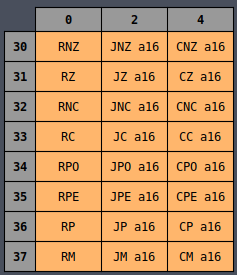Pattern: 8’o3?0, 8’o3?2, 8’o3?4

opcode[2:0]

• 000 - Conditional Return
• 010 - Conditional Jump
• 100 - Conditional Call

opcode[5:4]

• 00 - Zero Flag
• 01 - Carry Flag
• 10 - Parity Flag
• 11 - Sign Flag

opcode

• 0 - Unset
• 1 - Set
 `````` 1 2 3 4 5 6 7 8 9 10 11 12 13 14 15 16 17 18 19 20 21 22 23 24 25 26 27 28 29 30 31 32 33 34 35 36 37 38 39 40 41 42 43 44 45 46 47 48 49 50 51 52 53 54 55 56 57 58 59 60 61 62 63 64 65 66 67 68 69 70 71 72 73 74 75 76 77 78 79 80 81 82 83 84 85 86 87 88 89 90 91 92 93 94 95 96 97 98 99 100 101 102 103 104 105 106 107 108 109 110 111 112 113 114 115 116 117 118 119 120 121 122 123 124 125 126 127 128 129 130 131 132 133 134 135 136 137 138 139 `````` ``````// Jump Conditional // opcode[5:4] - flag // opcode - set (1) / unset (0) 8'o3?2: begin if (stage == 3) begin if (flags[opcode[5:4]] != opcode) begin ctrl_word[REG_WR_SEL4:REG_WR_SEL0] = REG_PC; ctrl_word[REG_EXT1:REG_EXT0] = REG_INC2; stage_rst = 1'b1; end else begin ctrl_word[REG_RD_SEL4:REG_RD_SEL0] = REG_PC; ctrl_word[REG_OE] = 1'b1; ctrl_word[MEM_MAR_WE] = 1'b1; end end else if (stage == 4) begin ctrl_word[REG_WR_SEL4:REG_WR_SEL0] = REG_WZ_Z; ctrl_word[REG_WE] = 1'b1; ctrl_word[MEM_OE] = 1'b1; end else if (stage == 5) begin ctrl_word[REG_WR_SEL4:REG_WR_SEL0] = REG_PC; ctrl_word[REG_EXT1:REG_EXT0] = REG_INC; end else if (stage == 6) begin ctrl_word[REG_RD_SEL4:REG_RD_SEL0] = REG_PC; ctrl_word[REG_OE] = 1'b1; ctrl_word[MEM_MAR_WE] = 1'b1; end else if (stage == 7) begin ctrl_word[REG_WR_SEL4:REG_WR_SEL0] = REG_WZ_W; ctrl_word[REG_WE] = 1'b1; ctrl_word[MEM_OE] = 1'b1; end else if (stage == 8) begin ctrl_word[REG_WR_SEL4:REG_WR_SEL0] = REG_PC; ctrl_word[REG_RD_SEL4:REG_RD_SEL0] = REG_WZ; ctrl_word[REG_WE] = 1'b1; ctrl_word[REG_OE] = 1'b1; stage_rst = 1'b1; end end // Call Conditional // opcode[5:4] - flag // opcode - set (1) / unset (0) 8'o3?4: begin if (stage == 3) begin if (flags[opcode[5:4]] != opcode) begin ctrl_word[REG_WR_SEL4:REG_WR_SEL0] = REG_PC; ctrl_word[REG_EXT1:REG_EXT0] = REG_INC2; stage_rst = 1'b1; end else begin ctrl_word[REG_RD_SEL4:REG_RD_SEL0] = REG_PC; ctrl_word[REG_OE] = 1'b1; ctrl_word[MEM_MAR_WE] = 1'b1; end end else if (stage == 4) begin ctrl_word[REG_WR_SEL4:REG_WR_SEL0] = REG_WZ_Z; ctrl_word[REG_WE] = 1'b1; ctrl_word[MEM_OE] = 1'b1; end else if (stage == 5) begin ctrl_word[REG_WR_SEL4:REG_WR_SEL0] = REG_PC; ctrl_word[REG_EXT1:REG_EXT0] = REG_INC; end else if (stage == 6) begin ctrl_word[REG_RD_SEL4:REG_RD_SEL0] = REG_PC; ctrl_word[REG_OE] = 1'b1; ctrl_word[MEM_MAR_WE] = 1'b1; end else if (stage == 7) begin ctrl_word[REG_WR_SEL4:REG_WR_SEL0] = REG_WZ_W; ctrl_word[REG_WE] = 1'b1; ctrl_word[MEM_OE] = 1'b1; end else if (stage == 8) begin ctrl_word[REG_WR_SEL4:REG_WR_SEL0] = REG_PC; ctrl_word[REG_EXT1:REG_EXT0] = REG_INC; end else if (stage == 9) begin ctrl_word[REG_WR_SEL4:REG_WR_SEL0] = REG_SP; ctrl_word[REG_EXT1:REG_EXT0] = REG_DEC; end else if (stage == 10) begin ctrl_word[REG_RD_SEL4:REG_RD_SEL0] = REG_SP; ctrl_word[REG_OE] = 1'b1; ctrl_word[MEM_MAR_WE] = 1'b1; end else if (stage == 11) begin ctrl_word[REG_RD_SEL4:REG_RD_SEL0] = REG_PC_C; ctrl_word[REG_OE] = 1'b1; ctrl_word[MEM_WE] = 1'b1; end else if (stage == 12) begin ctrl_word[REG_WR_SEL4:REG_WR_SEL0] = REG_SP; ctrl_word[REG_EXT1:REG_EXT0] = REG_DEC; end else if (stage == 13) begin ctrl_word[REG_RD_SEL4:REG_RD_SEL0] = REG_SP; ctrl_word[REG_OE] = 1'b1; ctrl_word[MEM_MAR_WE] = 1'b1; end else if (stage == 14) begin ctrl_word[REG_RD_SEL4:REG_RD_SEL0] = REG_PC_P; ctrl_word[REG_OE] = 1'b1; ctrl_word[MEM_WE] = 1'b1; end else if (stage == 15) begin ctrl_word[REG_RD_SEL4:REG_RD_SEL0] = REG_WZ; ctrl_word[REG_WR_SEL4:REG_WR_SEL0] = REG_PC; ctrl_word[REG_WE] = 1'b1; ctrl_word[REG_OE] = 1'b1; stage_rst = 1'b1; end end // Return Conditional // opcode[5:4] - flag // opcode - set (1) / unset (0) 8'o3?0: begin if (stage == 3) begin if (flags[opcode[5:4]] != opcode) begin stage_rst = 1'b1; end else begin ctrl_word[REG_RD_SEL4:REG_RD_SEL0] = REG_SP; ctrl_word[REG_OE] = 1'b1; ctrl_word[MEM_MAR_WE] = 1'b1; end end else if (stage == 4) begin ctrl_word[REG_WR_SEL4:REG_WR_SEL0] = REG_WZ_W; ctrl_word[REG_WE] = 1'b1; ctrl_word[MEM_OE] = 1'b1; end else if (stage == 5) begin ctrl_word[REG_WR_SEL4:REG_WR_SEL0] = REG_SP; ctrl_word[REG_EXT1:REG_EXT0] = REG_INC; end else if (stage == 6) begin ctrl_word[REG_RD_SEL4:REG_RD_SEL0] = REG_SP; ctrl_word[REG_OE] = 1'b1; ctrl_word[MEM_MAR_WE] = 1'b1; end else if (stage == 7) begin ctrl_word[REG_WR_SEL4:REG_WR_SEL0] = REG_WZ_Z; ctrl_word[REG_WE] = 1'b1; ctrl_word[MEM_OE] = 1'b1; end else if (stage == 8) begin ctrl_word[REG_WR_SEL4:REG_WR_SEL0] = REG_SP; ctrl_word[REG_EXT1:REG_EXT0] = REG_INC; end else if (stage == 9) begin ctrl_word[REG_RD_SEL4:REG_RD_SEL0] = REG_WZ; ctrl_word[REG_WR_SEL4:REG_WR_SEL0] = REG_PC; ctrl_word[REG_WE] = 1'b1; ctrl_word[REG_OE] = 1'b1; stage_rst = 1'b1; end end ``````

#### Push / Pop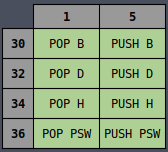Pattern: 8’o301, 8’o321, 8’o341, 8’o361, 8’o3?5

opcode[5:4]

• 000 - B
• 010 - D
• 100 - H
• 110 - PSW

opcode[2:0]

• 001 - POP
• 101 - PUSH
 `````` 1 2 3 4 5 6 7 8 9 10 11 12 13 14 15 16 17 18 19 20 21 22 23 24 25 26 27 28 29 30 31 32 33 34 35 36 37 38 39 40 41 42 43 44 45 46 47 48 49 50 51 52 53 54 55 56 57 58 59 60 61 62 63 64 65 66 67 68 69 70 71 72 73 74 75 76 `````` ``````// PUSH Rs // opcode[5:4] - Extended Register 8'o3?5: begin if (stage == 3) begin ctrl_word[REG_WR_SEL4:REG_WR_SEL0] = REG_SP; ctrl_word[REG_EXT1:REG_EXT0] = REG_DEC; end else if (stage == 4) begin ctrl_word[REG_RD_SEL4:REG_RD_SEL0] = REG_SP; ctrl_word[REG_OE] = 1'b1; ctrl_word[MEM_MAR_WE] = 1'b1; end else if (stage == 5) begin if (opcode[5:4] == 2'b11) begin // PSW ctrl_word[ALU_OE] = 1'b1; end else begin ctrl_word[REG_RD_SEL4:REG_RD_SEL0] = {2'b0, opcode[5:4], 1'b0}; ctrl_word[REG_OE] = 1'b1; end ctrl_word[MEM_WE] = 1'b1; end else if (stage == 6) begin ctrl_word[REG_WR_SEL4:REG_WR_SEL0] = REG_SP; ctrl_word[REG_EXT1:REG_EXT0] = REG_DEC; end else if (stage == 7) begin ctrl_word[REG_RD_SEL4:REG_RD_SEL0] = REG_SP; ctrl_word[REG_OE] = 1'b1; ctrl_word[MEM_MAR_WE] = 1'b1; end else if (stage == 8) begin if (opcode[5:4] == 2'b11) begin // PSW ctrl_word[ALU_FLAGS_OE] = 1'b1; end else begin ctrl_word[REG_RD_SEL4:REG_RD_SEL0] = {2'b0, opcode[5:4], 1'b1}; ctrl_word[REG_OE] = 1'b1; end ctrl_word[MEM_WE] = 1'b1; stage_rst = 1'b1; end end // POP Rs // opcode[5:4] - Extended Register 8'o301, 8'o321, 8'o341, 8'o361: begin if (stage == 3) begin ctrl_word[REG_RD_SEL4:REG_RD_SEL0] = REG_SP; ctrl_word[REG_OE] = 1'b1; ctrl_word[MEM_MAR_WE] = 1'b1; end else if (stage == 4) begin if (opcode[5:4] == 2'b11) begin // PSW ctrl_word[ALU_FLAGS_WE] = 1'b1; end else begin ctrl_word[REG_WR_SEL4:REG_WR_SEL0] = {2'b0, opcode[5:4], 1'b1}; ctrl_word[REG_WE] = 1'b1; end ctrl_word[MEM_OE] = 1'b1; end else if (stage == 5) begin ctrl_word[REG_WR_SEL4:REG_WR_SEL0] = REG_SP; ctrl_word[REG_EXT1:REG_EXT0] = REG_INC; end else if (stage == 6) begin ctrl_word[REG_RD_SEL4:REG_RD_SEL0] = REG_SP; ctrl_word[REG_OE] = 1'b1; ctrl_word[MEM_MAR_WE] = 1'b1; end else if (stage == 7) begin if (opcode[5:4] == 2'b11) begin // PSW ctrl_word[ALU_A_WE] = 1'b1; end else begin ctrl_word[REG_WR_SEL4:REG_WR_SEL0] = {2'b0, opcode[5:4], 1'b0}; ctrl_word[REG_WE] = 1'b1; end ctrl_word[MEM_OE] = 1'b1; end else if (stage == 8) begin ctrl_word[REG_WR_SEL4:REG_WR_SEL0] = REG_SP; ctrl_word[REG_EXT1:REG_EXT0] = REG_INC; stage_rst = 1'b1; end ``````

### SHLD / LHLD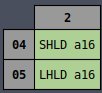Pattern: 8’o042, 8’o052

 `````` 1 2 3 4 5 6 7 8 9 10 11 12 13 14 15 16 17 18 19 20 21 22 23 24 25 26 27 28 29 30 31 32 33 34 35 36 37 38 39 40 41 42 43 44 45 46 47 48 49 50 51 52 53 54 55 56 57 58 59 60 `````` ``````// SHLD, LHLD // opcode - SHLD (0) / LHLD (1) 8'o042, 8'o052: begin if (stage == 3) begin ctrl_word[REG_RD_SEL4:REG_RD_SEL0] = REG_PC; ctrl_word[REG_OE] = 1'b1; ctrl_word[MEM_MAR_WE] = 1'b1; end else if (stage == 4) begin ctrl_word[MEM_OE] = 1'b1; ctrl_word[REG_WE] = 1'b1; ctrl_word[REG_WR_SEL4:REG_WR_SEL0] = REG_WZ_Z; end else if (stage == 5) begin ctrl_word[REG_WR_SEL4:REG_WR_SEL0] = REG_PC; ctrl_word[REG_EXT1:REG_EXT0] = REG_INC; end else if (stage == 6) begin ctrl_word[REG_RD_SEL4:REG_RD_SEL0] = REG_PC; ctrl_word[REG_OE] = 1'b1; ctrl_word[MEM_MAR_WE] = 1'b1; end else if (stage == 7) begin ctrl_word[MEM_OE] = 1'b1; ctrl_word[REG_WE] = 1'b1; ctrl_word[REG_WR_SEL4:REG_WR_SEL0] = REG_WZ_W; end else if (stage == 8) begin ctrl_word[REG_WR_SEL4:REG_WR_SEL0] = REG_PC; ctrl_word[REG_EXT1:REG_EXT0] = REG_INC; end else if (stage == 9) begin ctrl_word[REG_RD_SEL4:REG_RD_SEL0] = REG_WZ; ctrl_word[REG_OE] = 1'b1; ctrl_word[MEM_MAR_WE] = 1'b1; end else if (stage == 10) begin if (opcode == 1'b0) begin ctrl_word[REG_RD_SEL4:REG_RD_SEL0] = REG_HL_H; ctrl_word[REG_OE] = 1'b1; ctrl_word[MEM_WE] = 1'b1; end else begin ctrl_word[REG_WR_SEL4:REG_WR_SEL0] = REG_HL_H; ctrl_word[REG_WE] = 1'b1; ctrl_word[MEM_OE] = 1'b1; end end else if (stage == 11) begin ctrl_word[REG_WR_SEL4:REG_WR_SEL0] = REG_WZ; ctrl_word[REG_EXT1:REG_EXT0] = REG_INC; end else if (stage == 12) begin ctrl_word[REG_RD_SEL4:REG_RD_SEL0] = REG_WZ; ctrl_word[REG_OE] = 1'b1; ctrl_word[MEM_MAR_WE] = 1'b1; end else if (stage == 13) begin if (opcode == 1'b0) begin ctrl_word[REG_RD_SEL4:REG_RD_SEL0] = REG_HL_L; ctrl_word[REG_OE] = 1'b1; ctrl_word[MEM_WE] = 1'b1; end else begin ctrl_word[REG_WR_SEL4:REG_WR_SEL0] = REG_HL_L; ctrl_word[REG_WE] = 1'b1; ctrl_word[MEM_OE] = 1'b1; end stage_rst = 1'b1; end end ``````

### NOP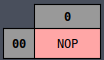Pattern: 8’o000

 ``````1 2 3 4 `````` ``````// NOP 8'o000: begin stage_rst = 1'b1; end ``````

### HLT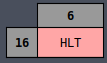Pattern: 8’o166

 ``````1 2 3 4 5 6 `````` ``````// HLT 8'o166: begin if (stage == 3) begin ctrl_word[HLT] = 1'b1; end end ``````

### OUT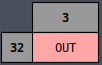Pattern: 8’o323

 ``````1 2 3 4 5 6 7 8 9 `````` ``````// OUT 8'o323: begin if (stage == 3) begin ctrl_word[REG_WR_SEL4:REG_WR_SEL0] = REG_PC; ctrl_word[REG_EXT1:REG_EXT0] = REG_INC; ctrl_word[DISPLAY] = 1'b1; stage_rst = 1'b1; end end ``````

## Results

To prove that things are working as expected, I wrote a simple program that exercises many (but not all) of the instructions. I tested all of the instructions individually in simulation, but it would be difficult to write a program that used every instruction. Or at least I can’t think of one.

 `````` 1 2 3 4 5 6 7 8 9 10 11 12 13 14 15 16 17 18 19 20 21 22 23 24 25 26 27 28 29 30 31 32 33 34 35 `````` ``````lxi sp, \$f0 mvi a, \$1 mvi b, \$0 loop: out mov c, a mov a, b cpi \$1 mov a, c jz rotate_right jnz rotate_left jmp loop rotate_right: rar cpi \$01 cz set_left jmp loop rotate_left: ral cpi \$80 cz set_right jmp loop set_left: mvi b, \$0 ret set_right: mvi b, \$1 ret hlt ``````

It uses the stack, jumps around unconditionally and conditionally, calls some functions unconditionally and conditionally, moves data around registers, uses immediate mode, and does some ALU operations. A nice variety of instructions (but not all).

The SAP-3 is opcode-compatible with the 8085 so we can use an 8080 Assembler to turn the assembly into machine code. All assembled it looks like this:

 ``````1 2 3 4 5 6 `````` ``````31 f0 00 3e 01 06 00 d3 ff 4f 78 fe 01 79 ca 17 00 c2 20 00 c3 07 00 1f fe 01 cc 29 00 c3 07 00 17 fe 80 cc 2c 00 c3 07 00 06 00 c9 06 01 c9 76 ``````

Behold, the world’s most complicated way to generate a Cylon eye effect:

## Source Code

The full unabridged Verilog code can be found here.